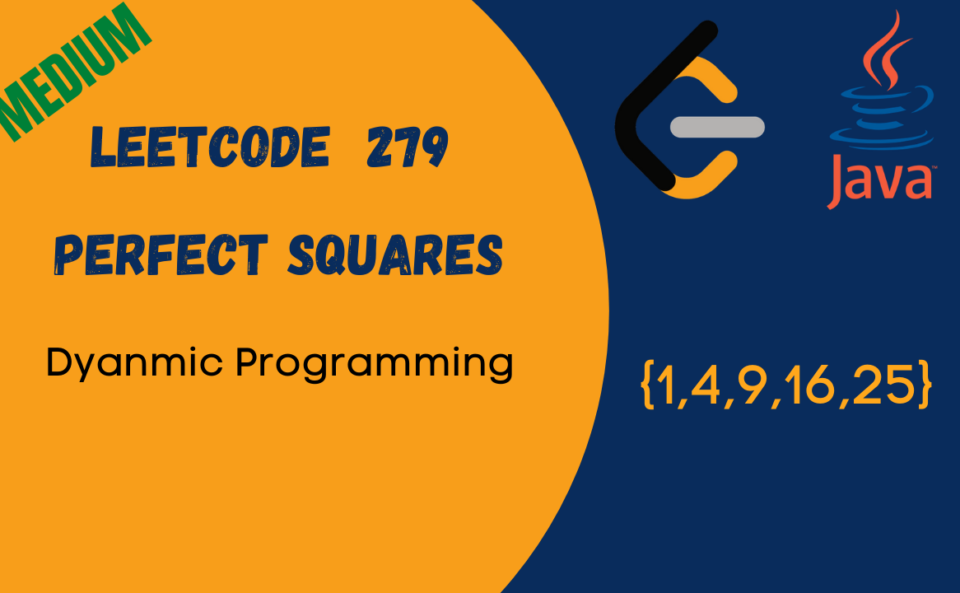# Leetcode Perfect Squares Java SolutionGiven a positive integer n, find the least number of perfect square numbers (for example, `1, 4, 9, 16, ...`) which sum to n.

Example 1:

```Input: n = `12`
Output: 3
Explanation: `12 = 4 + 4 + 4.````

Example 2:

```Input: n = `13`
Output: 2
Explanation: `13 = 4 + 9.````

Solution: We will solve this problem using Dynamic Programming, the sum of perfect squares for the number would be the number of perfect squares for previous number +1 whichever is minimum Math.min(dp[i], dp[i-j*j]+1)

``````class HackerHeap {
public int numSquares(int n) {
int max = (int) Math.sqrt(n);
int[] dp = new int[n+1];

Arrays.fill(dp, Integer.MAX_VALUE);

for(int i=1; i<=n;i++){
for(int j=1; j<=max;j++){
if(i==j*j) {
dp[i] = 1;
}else if(i>j*j){
dp[i] = Math.min(dp[i], dp[i-j*j]+1);
}else if(i<j*j) {
break;
}
}
}
return dp[n];
}
}``````